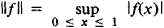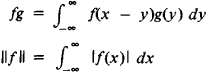# Normed Ring

## Normed Ring

an important concept of functional analysis that greatly extends the range of applications of functional analysis. The elements of a normed ring are at the same time the points of a certain geometric structure—a complete normed vector space—and the elements of a certain algebraic structure—a ring—in which multiplication by numbers is defined, with the algebraic operations being continuous with respect to the norm. Some examples of normed rings are the ring C of all continuous functions on the closed interval [0,1] with the usual algebraic operations and with normthe ring of matrices of order n, the ring of bounded operators on Hilbert space, and the ring L1 of all functions that are absolutely integrable on the line with multiplication defined as convolutionThe most highly developed of these theories is that of commutative normed rings, that is, normed rings in which multiplication is commutative: xy = yx. This theory was developed by I. M. Gel’fand.

Normed rings are also called Banach algebras.

### REFERENCE

Naimark, M. A. Normirovannye kol’lsa. Moscow, 1956.
References in periodicals archive ?
In this paper, we introduced NT normed ring space and we give properties of NT normed ring space.
If [parallel]x[parallel] : NTR [right arrow] [R.sup.+] U{0} function satisfies following condition; [parallel]x[parallel] is called NT normed ring on (NTR,*,#).
Now we show that (P(X), *, [intersection]), [parallel], [parallel]) is a NT normed ring space such that [parallel]A[parallel] = s(A).
It is clear that NT normed ring spaces are generally different from classical normed ring spaces since for neut(x) different from classical unit element.
Let (NTR, *, #), [parallel] x [parallel]) be a NT normed ring space.
Let ((NTR, *, #), [parallel]x[parallel]) be a NT normed ring space.
Let (NTR*,#), [parallel], [parallel]) be a NT normed ring space, {[X.sub.n]} be a sequence in this space.
Let (NTR*,#), [parallel] x [parallel]) be a NT normed ring space, {[x.sub.n]} be a sequence in this space.
Let (NTR*,#), [parallel]x[parallel]) be a NT normed ring space, {[x.sub.n]} be a sequence in this space.
Let (NTR *, #), [parallel] x [parallel]) be a NT normed ring space, {[X.sub.n]} be a sequence in this space.
Given a normed ring R, and a fixed element e in it, we could define the derivative D (f) of a map f : R [right arrow] R at an element r of R as
Naimark's book Normirovanie koltsa (Normed Rings) and I began to read it.

Site: Follow: Share:
Open / Close